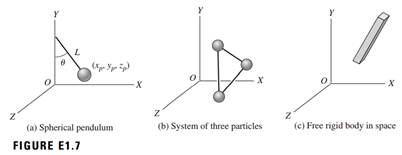### Create an Account

Home / Questions / Determine the number of degrees of freedom for the systems shown in Figure E17 Assume that...

# Determine the number of degrees of freedom for the systems shown in Figure E17 Assume that the length L of the pendulum shown in Figure E17a is constant and that the length between each pair of

Determine the number of degrees of freedom for the systems shown in Figure E1.7. Assume that the length L of the pendulum shown in Figure E1.7a is constant and that the length between each pair of particles in Figure E1.7b is constant.Jun 25 2020 View more View LessSubscribe To Get Solution